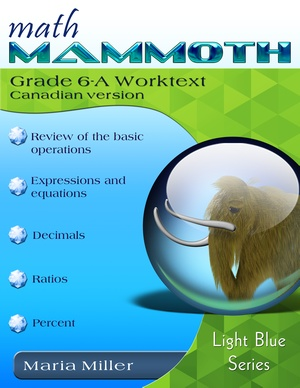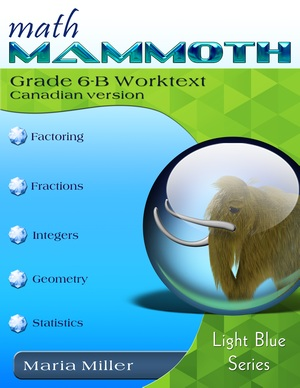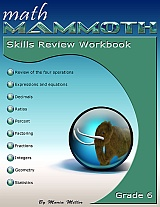^

Math Mammoth Grade 6 Canadian Version is a complete maths program for sixth grade, available both as digital and print versions.185 pages
162 lesson pages182 pages
157 lesson pages

## Prices & ordering

Grade 6 full set: USD \$42.50
(part A and B worktexts, answer keys,
tests, mixed revisions, worksheet
maker, and Soft-Pak)

Part 6-A only: USD \$21.25
(part A worktext, part A answer key,
tests, cumulative revisions, worksheet
maker, and Soft-Pak)

Part 6-B only: USD \$21.25
(part B worktext, part B answer key,
tests, cumulative revisions, worksheet
maker, and Soft-Pak)

#### Printed copies

You can purchase printed copies (perfect bound) at Lulu.

Tests & Cumulative Reviews 13.55 CAD

To go with the printed student books, you can purchase the digital version of the supportive materials: answer keys, tests, cumulative revisions, worksheet maker and software Soft-Pak (\$9.60 USD).

#### Skills Review WorkbookThis workbook is supplemental, and provides additional practice for the topics in the curriculum. I recommend you only purchase it once you are sure the student does need supplemental practice. Learn more.

At the heart of the curriculum are two student worktexts (A and B), each covering about half a year of maths work. These worktexts contain all the instruction and the exercises in the same book — which makes lesson preparation a breeze. The worktexts are written directly to the student, and thus they allow many children (if they can read) to teach themselves and to learn directly from the books.

You will also get separate answer keys, chapter tests, additional cumulative revisions (I do recommend you use at least some of them), and a versatile worksheet maker (Internet access required) for those times when your child needs just a bit more practice.

This curriculum is essentially the same as the U.S. version of Math Mammoth Grade 6, only customised for the Canadian audience in these aspects:

• The curriculum uses the metric measurement units. Since Canadians do use some imperial units (e.g. miles, pounds) in some contexts, the curriculum does infrequently use them.
• The spelling conforms mostly to American English, taking into account a few key differences where Canadian English follows British English.
• Numbers are formatted with a space as a thousands separator, and a point as a decimal separator.
• Page (paper) size is Letter.

The US version of the curriculum is aligned to the Common Core Standards, which means this curriculum may not be properly aligned to the sixth grade standards in your province. However, you can probably find material for any missing topics in neighbouring grades, which means you can simply re-order the material to solve most incompatibilities between different standards.

## Features

• Math Mammoth focuses on conceptual understanding. It explains the "WHY", so your children can understand the maths, not just learn "HOW" to do it.
• Concepts are often explained with visual models, followed by exercises using those models. These visual models can take the place of manipulatives for many children; however, it is very easy to add corresponding manipulatives to the lessons if so desired.
• The curriculum is mastery-oriented. This means it concentrates fairly long on a topic, delving into its various aspects. This promotes conceptual understanding, as opposed to spiral curricula that often tend to jump from topic to topic too much.
• There is a strong emphasis on mental math and number sense.
• It requires very little teacher preparation, which is a big help to most parents.• The curriculum has no separate teacher's manual nor is it scripted. The introduction to each chapter has some notes for the teacher concerning the material in the chapter. All the instruction is written directly to the student in the worktext, and there also exist accompanying videos where you can see Maria herself teach the material.
• After each chapter introduction, you will find a list of Internet links and resources (games, quizzes, animations, etc.) that can be used for fun, illustrations and further practice.

• The PDF files can be filled in on a computer, phone, or tablet, using the annotation tools found in many PDF apps. Learn more.
• BONUS! The download version also comes with BONUS Soft-Pak programs. The 6 programs in Soft-Pak (4 math, 1 language arts, 1 list maker) offer both on-screen and printable activities in a low-graphic, high content format. Read more and see screenshots.

## Overview of topics

The main areas of study in Math Mammoth Grade 6 are:

• revision of the basic operations with whole numbers
• beginning algebra topics: expressions, equations and inequalities
• revision of all decimal arithmetic
• introduction to ratios and percent
• prime factorisation, GCF, and LCM
• a revision of fraction arithmetic from 5th grade, plus a focus on division of fractions
• the concept of integers, coordinate grid, addition & subtraction of integers
• geometry: review of quadrilaterals & drawing problems; area of triangles and polygons; volume of rectangular prisms with fractional edge lengths; surface area
• statistics: concept of a distribution, measures of center, measures of variation, boxplots, histograms

Please also see the table of contents 6-A and 6-B (in the sample files), which will let you see the topics covered in more detail.

## Introduction

In sixth grade, students encounter the beginnings of algebra, learning about algebraic expressions, one-variable equations and inequalities, integers, and ratios. We also review and deepen the students' understanding of rational numbers: both fractions and decimals are studied in depth, while percent is a new topic for 6th grade. In geometry, students learn to compute the area of various polygons, and also calculate volume and surface area of various solids. The last major area of study is statistics, where students learn to summarize and describe distributions using both measures of center and variability.

The year starts out with a review of the four operations with whole numbers (including long division), place value, and rounding. Students are also introduced to exponents and do some problem solving.

Chapter 2 starts the study of algebra topics, delving first into expressions and equations. Students practice writing expressions in many different ways, and use properties of operations and the idea of maintaining the equality of both sides of an equation to solve simple one-step equations. We also study briefly inequalities and using two variables.

Chapter 3 has to do with decimals. This is a long chapter, as we review all of decimal arithmetic, just using more decimal digits than in 5th grade. Students also convert measuring units in this chapter.

Ratios is a new topic (chapter 4). Students are already familiar with finding fractional parts from earlier grades, and now it is time to advance that knowledge into the study of ratios, which arise naturally from dividing a quantity into many equal parts. We study such topics as rates, unit rates, equivalent ratios, and problem solving using bar models.

Percent (chapter 5) is an important topic to understand thoroughly, because of its many applications in real life. The goal of this chapter is to develop a basic understanding of percent, to see percentages as decimals, and to learn to calculate discounts.

In chapter 6, we first review prime factorization, and then study the greatest common factor and the least common multiple.

Chapter 7 first presents a thorough review of fraction operations from 5th grade (addition, subtraction, and multiplication). The topic of focus in the chapter is fraction division. Students also do some problem solving with fractions.

In chapter 8, students are introduced to integers. They plot points in all four quadrants of the coordinate plane and learn to add and subtract with negative numbers. Multiplication and division of integers are studied in 7th grade.

In geometry (chapter 9), the focus is on the area of polygons. This is studied in a logical progression: first, the area of right triangles, then the area of parallelograms, then, the area of triangles, and lastly the area of polygons. We also review some topics from earlier grades (quadrilaterals, perimeter, basic drawing). Other major topics in this chapter are calculating surface area of some solids using nets, and volume of rectangular prisms with fractional edge lengths.

Lastly, in chapter 10, students study statistics. The focus idea is that of a statistical distribution. Students learn about measures of center and measures of variability. They learn how to make dot plots, histograms, boxplots, and stem-and-leaf plots. All of these lessons are necessary to summarize and analyze distributions.

## Manipulatives

Here is a list of manipulatives, or actually just measurement tools, that are needed for grade 6.

1. A ruler that measures in inches
2. A ruler that measures in centimeters
3. A protractor.

## How and where to order

• As a digital version on this site (Mathmammoth.com) — using the "Add to cart" buttons.
• As a print version at Lulu. Follow the links from this site to Lulu.

Also available:

Get grades 1-3 in a discounted bundle — 35% off!

Get grades 4-7 in a discounted bundle — 35% off! This means you save \$60 USD (or the equivalent in your currency).

Note: The grades 4-7 bundle currently includes the Canadian version for grades 4-6, and the U.S. version for grade 7. We decided to offer this bundle prior to us publishing the grade 7 CA version, so that people can get the discount when purchasing a bundle.

The main possible issue is that the U.S. version of grade 7 uses customary units (inches, feet, miles, pounds, gallons) in several places. However, since Canadians are typically familiar with these, it will probably not pose a big problem.

By purchasing any of the books, permission IS granted for the purchaser (teacher or parent) to reproduce this material to be used with his/her students in a teaching situation; not for commercial resale. Please note: you are not permitted to share the material with other teachers or parents.

In other words, you are permitted to make copies for the students/children you are teaching, but not for other teachers' usage.

WAIT!

Receive my monthly collection of math tips & resources directly in your inbox — and get a FREE Math Mammoth book!You can unsubscribe at any time.

### Math Mammoth Tour

Confused about the different options? Take a virtual email tour around Math Mammoth! You'll receive:

An initial email to download your GIFT of over 400 free worksheets and sample pages from my books. Six other "TOURSTOP" emails that explain the important things and commonly asked questions concerning Math Mammoth curriculum. (Find out the differences between all these different-colored series!)

This way, you'll have time to digest the information over one or two weeks, plus an opportunity to ask me personally about the curriculum.
A monthly collection of math teaching tips & Math Mammoth updates (unsubscribe any time)### "Mini" Math Teaching Course

This is a little "virtual" 2-week course, where you will receive emails on important topics on teaching math, including:

- How to help a student who is behind
- Troubles with word problems
- Teaching multiplication tables
- Why fractions are so difficult
- The value of mistakes
- Should you use timed tests
- And more!

A GIFT of over 400 free worksheets and sample pages from my books right in the very beginning.A monthly collection of math teaching tips & Math Mammoth updates (unsubscribe any time)
Enter your email to receive math teaching tips, resources, Math Mammoth news & sales, humor, and more! I tend to send out these tips about once monthly, near the beginning of the month, but occasionally you may hear from me twice per month (and sometimes less often).• A GIFT of over 400 free worksheets and sample pages from my books.